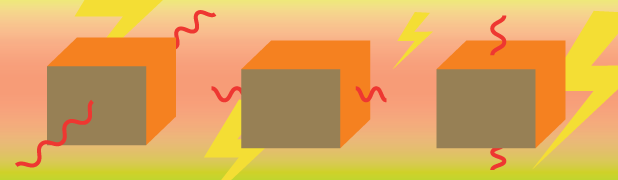# Circuit Diagrams Ignore The Orientation Of The Resistor. Is This An Oversight?A rectangular conducting homogeneous (which means the material is the same throughout) block has dimensions $1\text{ cm} \times2\text{ cm} \times3\text{ cm}$ . A voltage $V$ is applied between all opposite faces of the block and the corresponding currents were recorded. What is the maximum current measured in Amps if the minimum current recorded is $1\text{ mA}$?

×